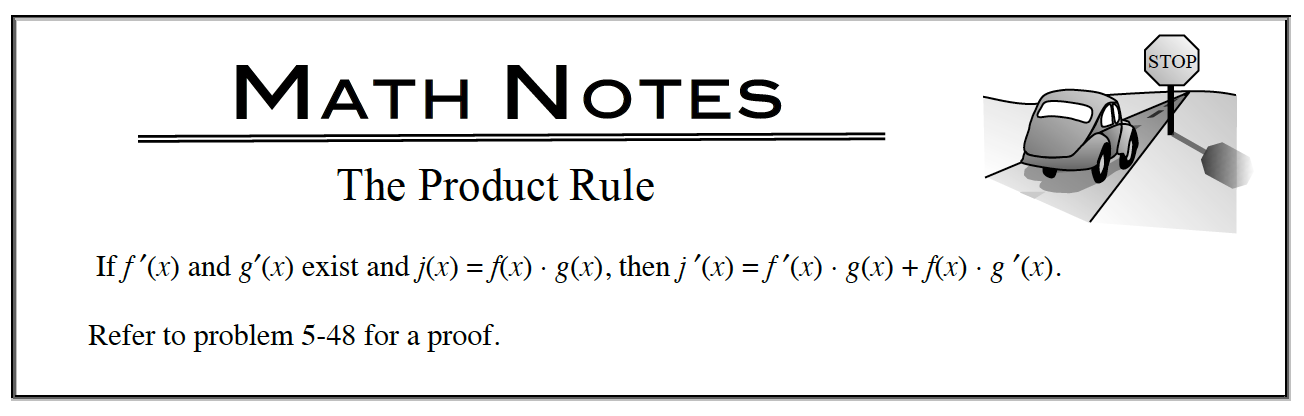### Home > APCALC > Chapter 5 > Lesson 5.2.1 > Problem5-55

5-55.

Draw the graph of $f(x) = \sqrt{x}\operatorname{cos}(x)$ for $0 ≤ x ≤ 3$. 5-55 HW eTool (Desmos). Homework Help ✎

1. Write an equation for $f^\prime$.2. Graph $y = f^\prime(x)$ using your graphing calculator. Then, use this graph to locate the $x$‑value of the local maximum of $f$ over $0 ≤ x ≤ 3$.

The location of a local maximum on $f\left(x\right)$ can be found where $f^\prime\left(x\right) = 0$ AND changes from positive values to negative values.

3. Likewise, use a graph of $y = f ^{\prime\prime}(x)$ to locate the point of inflection of $f$ for $0 ≤ x ≤ 3$.

A zero on the second derivative that crosses the $x$-axis (or changes sign) indicates a point of inflection on the original function.

Use the eTool below to help solve the problem.
Click the link at right for the full version of the eTool: Calc 5-55 HW eTool